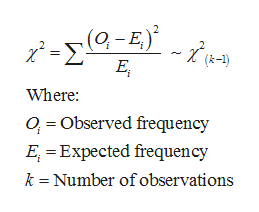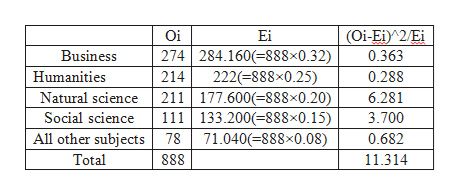# The director of library services at a college did a survey of types of books (by subject) in the circulation library. Then she used library records to take a random sample of 888 books checked out last term and classified the books in the sample by subject. The results are shown below.Subject AreaPercent of Books on Subject in Circulation Library on This SubjectNumber of Books in Sample on This SubjectBusiness32%274Humanities25%214Natural Science20%211Social Science15%111All other subjects8%78Using a 5% level of significance, test the claim that the subject distribution of books in the library fits the distribution of books checked out by students.(a) What is the level of significance? State the null and alternate hypotheses.H0: The distributions are the same.H1: The distributions are different.H0: The distributions are different.H1: The distributions are different.     H0: The distributions are different.H1: The distributions are the same.H0: The distributions are the same.H1: The distributions are the same.(b) Find the value of the chi-square statistic for the sample. (Round the expected frequencies to three decimal places. Round the test statistic to three decimal places.) Are all the expected frequencies greater than 5?YesNo    What sampling distribution will you use?Student's tnormal     chi-squarebinomialuniformWhat are the degrees of freedom? (c) Estimate the P-value of the sample test statistic. P-value > 0.1000.050 < P-value < 0.100     0.025 < P-value < 0.0500.010 < P-value < 0.0250.005 < P-value < 0.010P-value < 0.005(d) Based on your answers in parts (a) to (c), will you reject or fail to reject the null hypothesis of independence?Since the P-value > α, we fail to reject the null hypothesis.Since the P-value > α, we reject the null hypothesis.     Since the P-value ≤ α, we reject the null hypothesis.Since the P-value ≤ α, we fail to reject the null hypothesis.(e) Interpret your conclusion in the context of the application.At the 5% level of significance, the evidence is sufficient to conclude that the subject distribution of books in the library is different from that of books checked out by students.At the 5% level of significance, the evidence is insufficient to conclude that the subject distribution of books in the library is different from that of books checked out by students.

Question
34 views

The director of library services at a college did a survey of types of books (by subject) in the circulation library. Then she used library records to take a random sample of 888 books checked out last term and classified the books in the sample by subject. The results are shown below.

 Subject Area Percent of Books on Subject in Circulation Library on This Subject Number of Books in Sample on This Subject Business 32% 274 Humanities 25% 214 Natural Science 20% 211 Social Science 15% 111 All other subjects 8% 78

Using a 5% level of significance, test the claim that the subject distribution of books in the library fits the distribution of books checked out by students.

(a) What is the level of significance?

State the null and alternate hypotheses.
H0: The distributions are the same.
H1: The distributions are different.H0: The distributions are different.
H1: The distributions are different.     H0: The distributions are different.
H1: The distributions are the same.H0: The distributions are the same.
H1: The distributions are the same.

(b) Find the value of the chi-square statistic for the sample. (Round the expected frequencies to three decimal places. Round the test statistic to three decimal places.)

Are all the expected frequencies greater than 5?
YesNo

What sampling distribution will you use?
Student's tnormal     chi-squarebinomialuniform

What are the degrees of freedom?

(c) Estimate the P-value of the sample test statistic.
P-value > 0.1000.050 < P-value < 0.100     0.025 < P-value < 0.0500.010 < P-value < 0.0250.005 < P-value < 0.010P-value < 0.005

(d) Based on your answers in parts (a) to (c), will you reject or fail to reject the null hypothesis of independence?
Since the P-value > α, we fail to reject the null hypothesis.Since the P-value > α, we reject the null hypothesis.     Since the P-value ≤ α, we reject the null hypothesis.Since the P-value ≤ α, we fail to reject the null hypothesis.

(e) Interpret your conclusion in the context of the application.
At the 5% level of significance, the evidence is sufficient to conclude that the subject distribution of books in the library is different from that of books checked out by students.At the 5% level of significance, the evidence is insufficient to conclude that the subject distribution of books in the library is different from that of books checked out by students.
check_circle

Step 1

a)The level of significance is 5%.

The null and alternative hypotheses are stated below:

H0: The distributions are same.

Ha: The distributions are different.

b) The test statistic is given below:help_outlineImage Transcriptionclose(0,-E.) ΤΣΟΕ.. (k-1) E Where: 0, = Observed frequency E, = Expected frequency k = Number of observations fullscreen
Step 2

The Expected frequencies and test statistics c...help_outlineImage TranscriptioncloseOi Ei (Oi-Ei)^2/Ei 274 284.160(=888×0.32) Business 0.363 222(=888×0.25) 0.288 Humanities 214 211 177.600(=888x0.20) 111 133.200(=888x0.15) Natural science 6.281 3.700 Social science All other subjects 78 71.040(=888×0.08) 0.682 Total 888 11.314 fullscreen

### Want to see the full answer?

See Solution

#### Want to see this answer and more?

Solutions are written by subject experts who are available 24/7. Questions are typically answered within 1 hour.*

See Solution
*Response times may vary by subject and question.
Tagged in

### Other